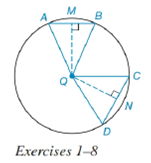Chapter 6.4, Problem 3EElementary Geometry For College St...

7th Edition
Alexander + 2 others
ISBN: 9781337614085

Solutions

Chapter
SectionElementary Geometry For College St...

7th Edition
Alexander + 2 others
ISBN: 9781337614085
Textbook Problem

In Exercises 1 to 8, use the figure provided.If m C D ⌢ < m A B ⌢ , write an inequality that compares Q M and Q N .To determine

To write:

An inequality that compares QM and QN.

Explanation

Given:

mCD<mAB and the figure given below

Theorem:

In a circle (or in congruent circles) containing two unequal minor arcs, the greater minor arc corresponds to the longer of the chords related to these arcs.

In a circle (or in congruent circles) containing two unequal chords, the shorter chord is at the greater distance from the center of the circle.

Calculation:

Using the theorem.

And since the arcs are unequal i.e. mCD<mAB and from the given figure,

The chord corresponding to arc mCD is CD and the chord corresponding to mAB is AB, we conclude that CD¯<AB¯

Still sussing out bartleby?

Check out a sample textbook solution.

See a sample solution

The Solution to Your Study Problems

Bartleby provides explanations to thousands of textbook problems written by our experts, many with advanced degrees!

Get Started

A population of N = 15 scores has SX = 120. What is the population mean?

Statistics for The Behavioral Sciences (MindTap Course List)

Evaluate the integral. 01(1+r)3dr

Calculus: Early Transcendentals

In problems 37-48, compute and simplify so that only positive exponents remain. 42.

Mathematical Applications for the Management, Life, and Social Sciences

Find the complex conjugate and the modulus of the number. 17. 4i

Single Variable Calculus: Early Transcendentals, Volume I

In Exercises 2340, find the indicated limit. 32. limx2(x2+1)(x24)

Applied Calculus for the Managerial, Life, and Social Sciences: A Brief Approach

Evaluate the integral. 042sds

Single Variable Calculus: Early Transcendentals

Find if , y = sin 2t.

Study Guide for Stewart's Single Variable Calculus: Early Transcendentals, 8th